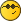A question on Pendulum experimentsolidMemberPosts: 169 Threads: 17 Joined: Nov 2019 Institution: Université de Bretagne Occidentale 12-24-2020, 03:56 AM (This post was last modified: 12-24-2020, 04:10 AM by solid.) x = A sin(omega t)                                      # deviation angle d sin(omega t) /dt= omega cos(omega t) v = dx/dt = (A omega) cos(omega t)          # rotation speed s = dA²omega²                                           # square standard deviation 'amplitude' calculated (dA² omega²)/omega/omega = dA² represents the standard deviation of the angle (for small harmonic oscillations)...i think. This example is too complicated for a tutorial... « Next Oldest | Next Newest »